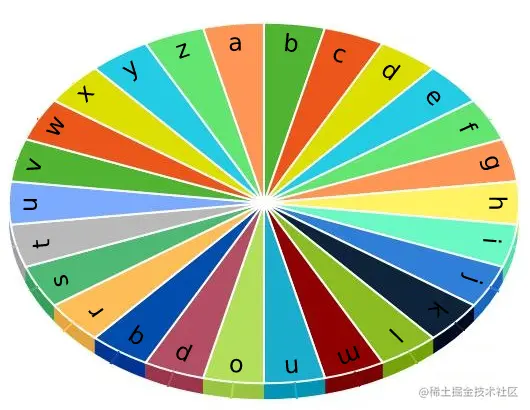# leetcode 1974. Minimum Time to Type Word Using Special Typewriter（python）

2022-01-29 15:56:13

This article has participated in 「 Digging force Star Program 」, Win a creative gift bag , Challenge creation incentive fund .

### describe

There is a special typewriter with lowercase English letters 'a' to 'z' arranged in a circle with a pointer. A character can only be typed if the pointer is pointing to that character. The pointer is initially pointing to the character 'a'.Each second, you may perform one of the following operations:

• Move the pointer one character counterclockwise or clockwise.
• Type the character the pointer is currently on.

Given a string word, return the minimum number of seconds to type out the characters in word.

Example 1:

``````Input: word = "abc"
Output: 5
Explanation:
The characters are printed as follows:
- Type the character 'a' in 1 second since the pointer is initially on 'a'.
- Move the pointer clockwise to 'b' in 1 second.
- Type the character 'b' in 1 second.
- Move the pointer clockwise to 'c' in 1 second.
- Type the character 'c' in 1 second.
Copy code ``````

Example 2:

``````Input: word = "bza"
Output: 7
Explanation:
The characters are printed as follows:
- Move the pointer clockwise to 'b' in 1 second.
- Type the character 'b' in 1 second.
- Move the pointer counterclockwise to 'z' in 2 seconds.
- Type the character 'z' in 1 second.
- Move the pointer clockwise to 'a' in 1 second.
- Type the character 'a' in 1 second.
Copy code ``````

Example 3:

``````Input: word = "zjpc"
Output: 34
Explanation:
The characters are printed as follows:
- Move the pointer counterclockwise to 'z' in 1 second.
- Type the character 'z' in 1 second.
- Move the pointer clockwise to 'j' in 10 seconds.
- Type the character 'j' in 1 second.
- Move the pointer clockwise to 'p' in 6 seconds.
- Type the character 'p' in 1 second.
- Move the pointer counterclockwise to 'c' in 13 seconds.
- Type the character 'c' in 1 second.
Copy code ``````

Note:

``````1 <= word.length <= 100
word consists of lowercase English letters.
Copy code ``````

### analysis

According to the meaning , An example is given 26 A disk of lowercase English letters in order , When the pointer points to a letter , Need to print this letter , At first, the pointer points to the letter a .

The disc can rotate clockwise or counterclockwise , We can do two operations at a time ：

• Turn the disc clockwise or counterclockwise , Let the pointer point to word A character in , After one character, it takes one second , Moving a few times takes a few seconds
• Print this character , It only needs 1 second

The title gives a word word , Let's calculate and print this word The minimum number of seconds required .

In fact, the problem is very simple , It's traversal word Each character in , Then find the minimum number of pointer moves from the previous character to the next character , You can find it clockwise or counterclockwise , Then add up all the pointer movement time and the printing time of letters . The key is to calculate the time clockwise and counterclockwise .

``````class Solution(object):
def minTimeToType(self, word):
"""
:type word: str
:rtype: int
"""
result = 0
for i, letter in enumerate(word):
if i == 0:
result += 1 + min(ord(letter)-ord('a'), 122-ord(letter)+1)
else:
# 122  yes  z  Of  ascii  code  ,97  yes  a  Of  ascii  code
if ord(letter) > ord(word[i-1]):
result += 1 + min(ord(letter)-ord(word[i-1]), 122-ord(letter)+1+ord(word[i-1])-97)
elif ord(letter) < ord(word[i-1]):
result += 1 + min(ord(word[i-1])-ord(letter), 122-ord(word[i-1])+1+ord(letter)-97)
else:
result += 1
return result

Copy code ``````

### Running results

``````Runtime: 41 ms, faster than 8.36% of Python online submissions for Minimum Time to Type Word Using Special Typewriter.
Memory Usage: 13.4 MB, less than 53.99% of Python online submissions for Minimum Time to Type Word Using Special Typewriter.
Copy code ``````

### analysis

In fact, the simpler way , Use greedy algorithm to solve .

``````class Solution(object):
def minTimeToType(self, word):
"""
:type word: str
:rtype: int
"""
s = "abcdefghojklmnopqrstuvwxyz"
result = 0
cur = "a"
for letter in word:
if cur == letter:
result += 1
continue
result += min(abs(ord(letter)-ord(cur)),26-abs(ord(letter)-ord(cur))) + 1
cur = letter
return result
Copy code ``````

### Running results

``````Runtime: 20 ms, faster than 73.38% of Python online submissions for Minimum Time to Type Word Using Special Typewriter.
Memory Usage: 13.5 MB, less than 53.99% of Python online submissions for Minimum Time to Type Word Using Special Typewriter.
Copy code ``````